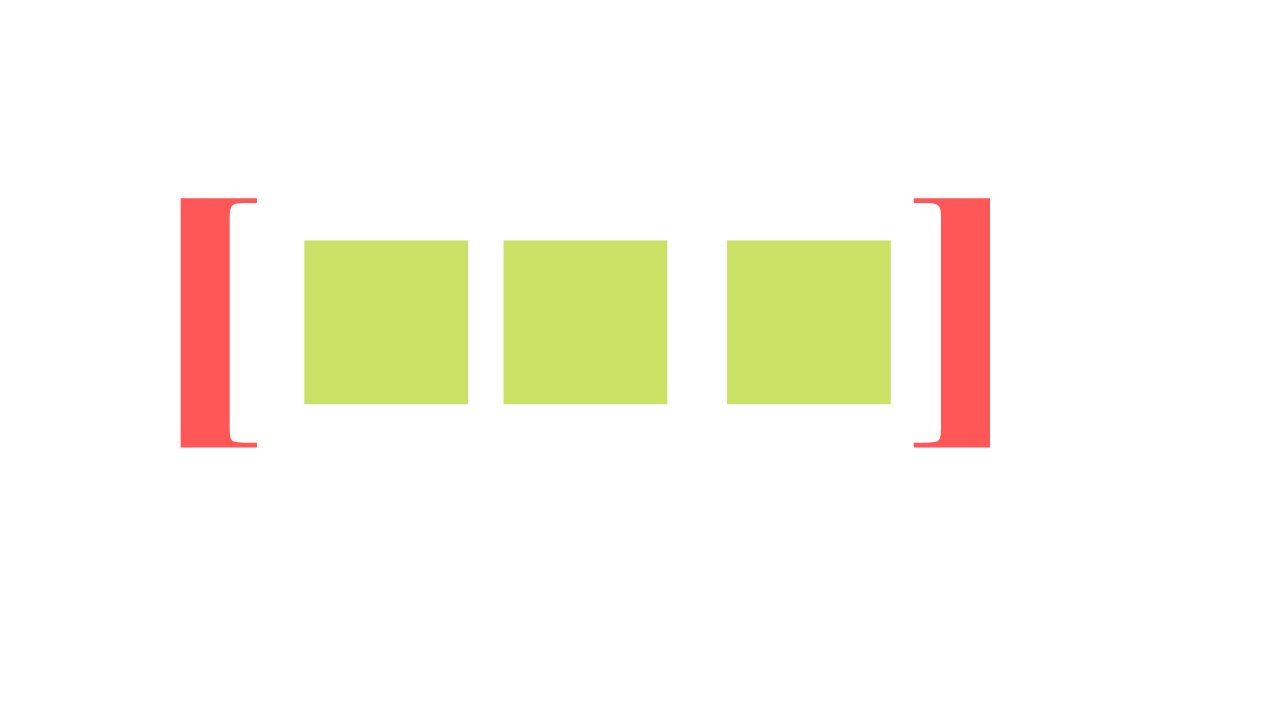# Arrays In Java With ExampleThe array is a data structure provided by Java which holds elements of the same data type with a fixed size.

An array can hold elements/ values of the same data type, an example being we can create an array that can store 100 elements of type either int/String/char etc.. We can not have array hold multiple datatypes like shown below.

Example 1: [1,2,3,4,5] | [‘a’,’b’,’c’,’d’] (correct)
Example 2: [1, ‘b’,3,’c’] (in correct)

### Array Declaration

dataType arrayName[] = new dataType[size];
OR
datatType arrayName[] = [value1, value2, value3];

dataType can be any data types like int, char, float, double, String, etc.. or can be a user-defined data type like a User object.

#### Example:

``int intArray[] = new int;``

In the above declaration, we declared an int array of size 10, which means it can hold 10 int values. similarly, the below declaration holds 50 char values.

``char charArray[] = new char;``

### Indexing

In an array, the index starts with 0 (zero), for example, let’s take the array

``int[] a = {4,3,2,1};``

a returns the first element in the array i.e 4. similarly a would return the second 3 and a would return the third element 2.

## Code Example

``````class ArrayExample {
public static void main(String [] args) {
int[] num = new int;
num = 4;
num = 5;
num = 7;
num = 9;
num = 3;

for(int i= 0; i<num.length; i++) {
System.out.println("Array element at index "+i+" = "+num[i]);
}
}
}``````

The resultant output for the above example would be

``````Array element at index 0 = 4
Array element at index 1 = 5
Array element at index 2 = 7
Array element at index 3 = 9
Array element at index 4 = 3``````

Here is the video explanation of an Array in Java.

Happy Coding!…